# Phoenix 常用函数

Phoenix 官方函数说明地址：https://phoenix.apache.org/language/functions.html

## 1. 数字函数

### 1.1 ROUND

``````ROUND(number)

ROUND(number, 2)

ROUND(timestamp)

ROUND(time, 'HOUR')

ROUND(date, 'MINUTE', 30)``````
``````select ROUND(1.6356);
select ROUND(1.6356,2);``````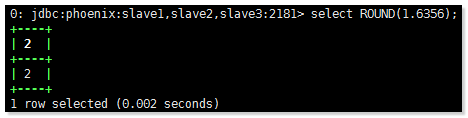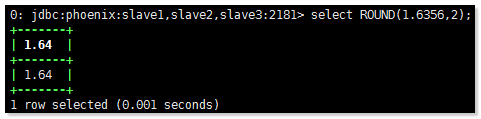1. CEIL

``````CEIL(number, 3)

CEIL(2.34)

CEIL(timestamp, 'SECOND', 30)

CEIL(date, 'DAY', 7)``````
``SELECT     CEIL(5.123456, 3);``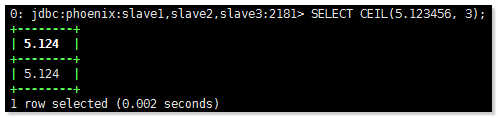``select     ROUND(TO_TIME('2005-10-01 14:03:22.559'), 'HOUR');``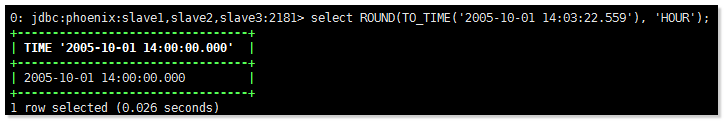### 1.2 FLOOR

``````FLOOR(timestamp)

FLOOR(date, 'DAY', 7)``````
``select     FLOOR(TO_date('2005-10-01 14:03:22.559'), 'DAY', 7);``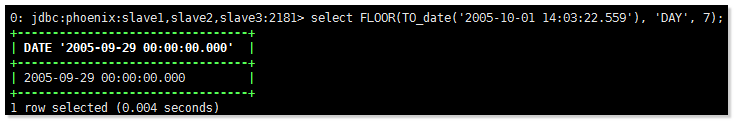### 1.3 TRUNC

``````TRUNC(timestamp, 'SECOND', 30)

TRUNC(date, 'DAY', 7)``````
``select TRUNC(TO_date('2005-10-01 14:03:22.559'), 'DAY', 7);``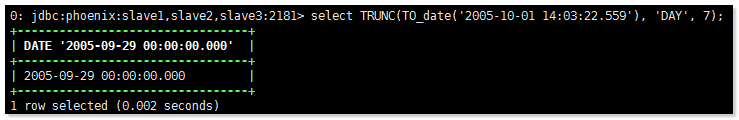### 1.4 TO_NUMBER

``TO_NUMBER('\$123.33', '\u00A4###.##')``

### 1.5 RAND

``````RAND()

RAND(5)``````
``select RAND(5);``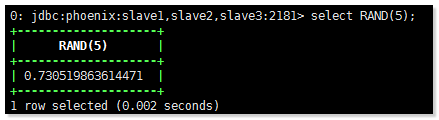## 2. 字符串函数

### 2.1 SUBSTR

``````SUBSTR('[Hello]', 2, 5)

SUBSTR('Hello World', -5)``````
``SELECT     SUBSTR('ABC',1,2);``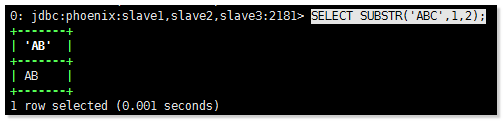### 2.2 INSTR

``````INSTR('Hello World', 'World')

INSTR('Simon says', 'mon')

INSTR('Peace on earth', 'war')``````
``select     INSTR('Hello World', 'World');``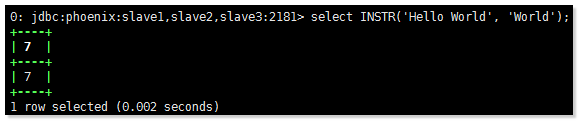### 2.3 TRIM

``TRIM('  Hello  ')``
``SELECT TRIM('  ABC      ');``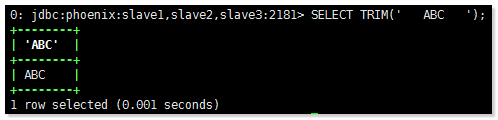### 2.4 LTRIM

``LTRIM('  Hello')``
``SELECT LTRIM('  ABC      ');``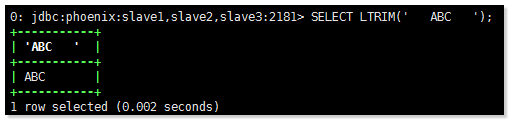### 2.5 RTRIM

``RTRIM('Hello  ')``
``SELECT RTRIM('  ABC      ');``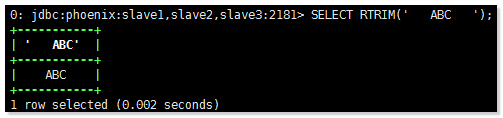``LPAD('John',30)``
``select     LPAD('John',30);``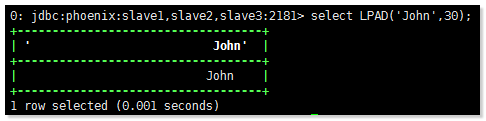### 2.7 LENGTH

``LENGTH('Hello')``
``select     LENGTH('abc');``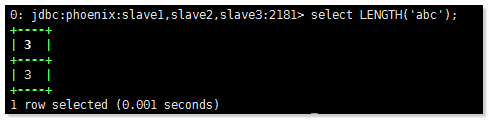### 2.8 REGEXP_SUBSTR

``REGEXP_SUBSTR('na1-appsrv35-sj35', '[^-]+') evaluates to 'na1'``
``select     REGEXP_SUBSTR('a1b2c3','\d');``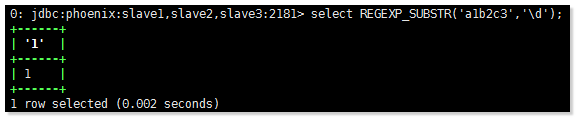### 2.9 REGEXP_REPLACE

``REGEXP_REPLACE('abc123ABC', '[0-9]+', '#') evaluates to 'abc#ABC'``
``select     REGEXP_REPLACE('a1b2c3','[0-9]','-');``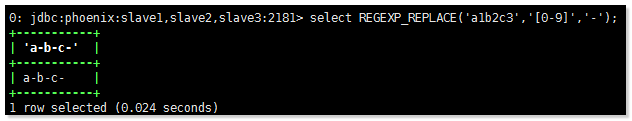### 2.10 REGEXP_SPLIT

VARCHAR ARRAY使用正则表达式将字符串拆分成一个。如果在正则表达式中具有特殊含义的字符将用作模式字符串中的常规定界符，则必须使用反斜线对其进行转义。

``````REGEXP_SPLIT('ONE,TWO,THREE', ',') evaluates to ARRAY['ONE', 'TWO', 'THREE']

REGEXP_SPLIT('ONE!#TWO#,!THREE', '[,!#]+') evaluates to ARRAY['ONE', 'TWO', 'THREE']``````
``select     REGEXP_SPLIT('ONE,TWO,THREE', ',');``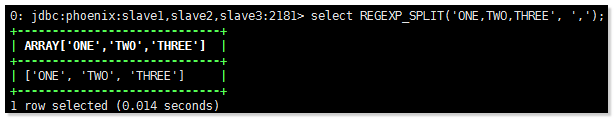### 2.11 UPPER

``UPPER('Hello')``
``SELECT     UPPER('AaBbCcDd');``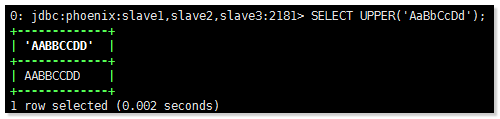### 2.12 LOWER

``LOWER('HELLO')``
``SELECT     LOWER('AaBbCcDd');``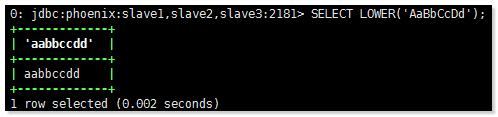### 2.13 REVERSE

``REVERSE('Hello')``
``SELECT     REVERSE('AaBbCcDd');``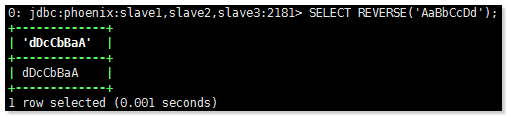### 2.14 TO_CHAR

``````TO_CHAR(myDate, '2001-02-03 04:05:06')

TO_CHAR(myDecimal, '#,##0.###')``````

## 3. 时间函数

### 3.1 TO_DATE

``````TO_DATE('Sat, 3 Feb 2001 03:05:06 GMT', 'EEE, d MMM yyyy HH:mm:ss z')

TO_DATE('1970-01-01', 'yyyy-MM-dd', 'GMT+1')``````
``select TO_DATE('2017-01-01',     'yyyy-MM-dd', 'GMT+1');``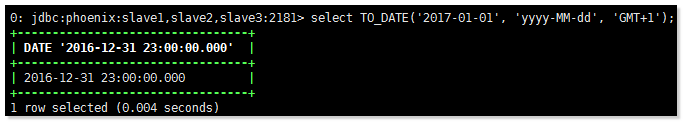### 3.2 TO_TIME

``````TO_TIME('2005-10-01 14:03:22.559')

TO_TIME('1970-01-01', 'yyyy-MM-dd', 'GMT+1')``````
``select     TO_TIME('2005-10-01 14:03:22.559');``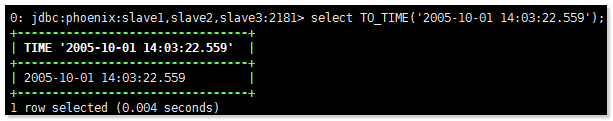### 3.3 TO_TIMESTAMP

``````TO_TIMESTAMP('2005-10-01 14:03:22.559')

TO_TIMESTAMP('1970-01-01', 'yyyy-MM-dd', 'GMT+1')``````
``select     TO_TIMESTAMP('2005-10-01 14:03:22.559');``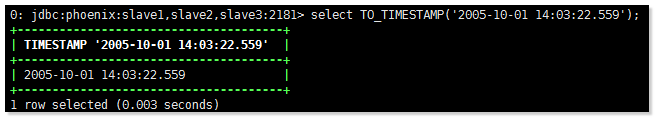### 3.4 CURRENT_TIME

``CURRENT_TIME()``
``select     CURRENT_TIME();``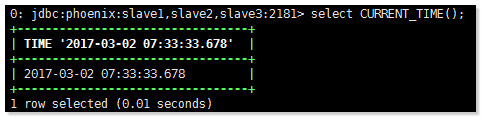### 3.5 CONVERT_TZ

``CONVERT_TZ(myDate, 'UTC', 'Europe/Prague')``
``select     CONVERT_TZ(TO_TIME('2005-10-01 14:03:22.559'), 'UTC', 'Europe/Prague');``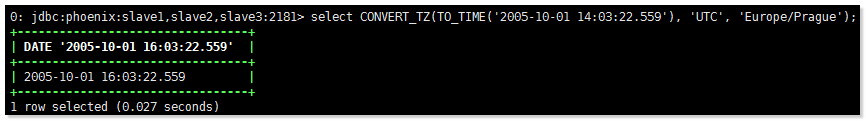### 3.6 TIMEZONE_OFFSET

``TIMEZONE_OFFSET('Indian/Cocos', myDate)``
``select     TIMEZONE_OFFSET('Indian/Cocos', TO_TIME('2005-10-01 14:03:22.559'));``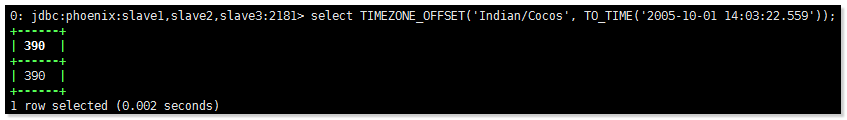### 3.7 NOW

``NOW()``
``select NOW();``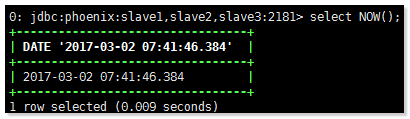### 3.8 YEAR

``YEAR(TO_DATE('2015-6-05'))``
``select     YEAR(TO_DATE('2015-6-05'));``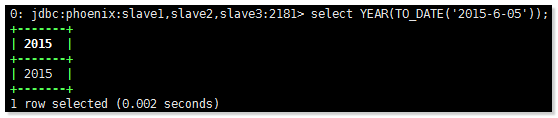### 3.9 MONTH

``MONTH(TO_TIMESTAMP('2015-6-05'))``
``select MONTH(TO_TIMESTAMP('2015-6-05'));``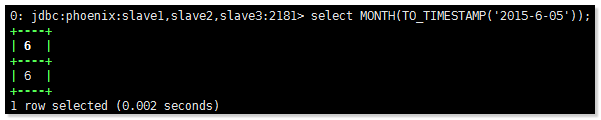### 3.10 WEEK

``WEEK(TO_TIME('2010-6-15'))``
``select WEEK(TO_TIME('2010-6-15'));``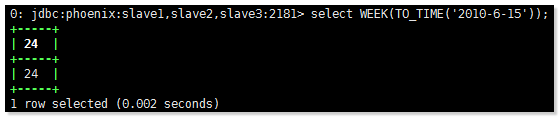### 3.11 DAYOFMONTH

``DAYOFMONTH(TO_DATE('2004-01-18 10:00:10'))``
``select DAYOFMONTH(TO_DATE('2004-01-18 10:00:10'));``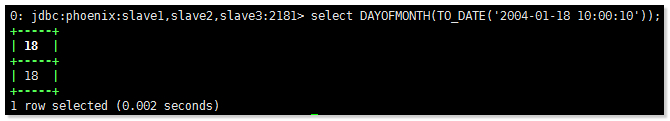### 3.12 HOUR

``HOUR(TO_TIMESTAMP('2015-6-05'))``
``select     HOUR(TO_TIMESTAMP('2015-6-05 12:12:12'));``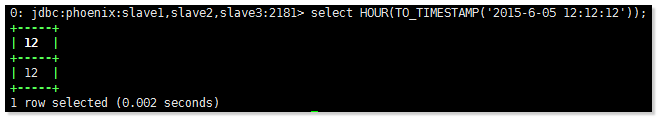### 3.13 MINUTE

``MINUTE(TO_TIME('2015-6-05'))``
``select MINUTE(TO_TIMESTAMP('2015-6-05     12:12:12'));``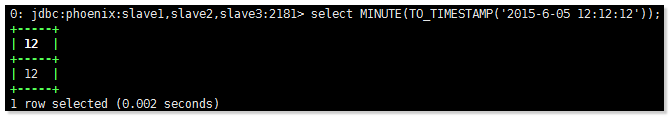### 3.14 SECOND

``SECOND(TO_DATE('2015-6-05'))``
``select SECOND(TO_TIMESTAMP('2015-6-05 12:13:14'));``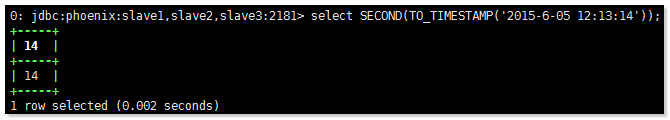## 4. 聚合函数

### 4.1AVG

``AVG()``
``select avg(CORE)     from WEB_STAT;``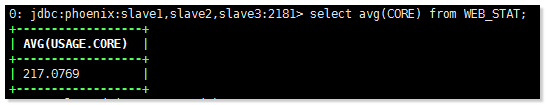### 4.2 COUNT

``COUNT(*)``
``select count(CORE)     from WEB_STAT;``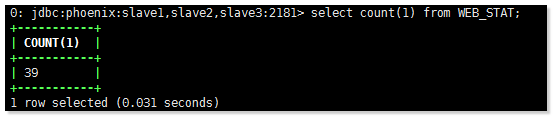### 4.3 MAX

``MAX(NAME)``
``select max(CORE)     from WEB_STAT;``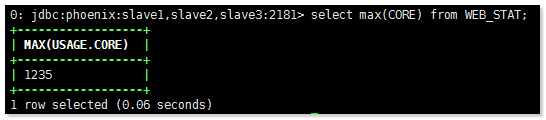### 4.4 MIN

MIN ( term )

``MIN(NAME)``
``select min(CORE) from     WEB_STAT;``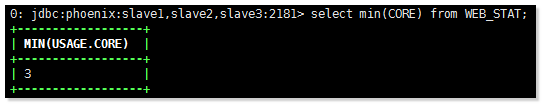### 4.5 SUM

SUM ( numericTerm )

``SUM(X)``
`` select sum(CORE)     from WEB_STAT;``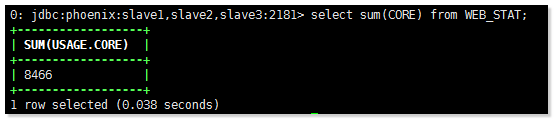### 4.6 PERCENTILE_CONT

``PERCENTILE_CONT( 0.9 ) WITHIN GROUP (ORDER BY X ASC)``
``select     PERCENTILE_CONT( 0.9 ) WITHIN GROUP (ORDER BY CORE ASC) from WEB_STAT;``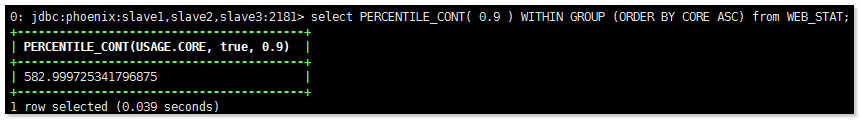### 4.7 PERCENTILE_DISC

PERCENTILE_DISC是假定离散分布模型的逆分布函数。它需要百分点值和排序规范，并从集合中返回一个元素。在计算中忽略空值。

``PERCENTILE_DISC( 0.9 ) WITHIN GROUP (ORDER BY X DESC)``
``select     PERCENTILE_DISC( 0.9 ) WITHIN GROUP (ORDER BY CORE DESC) from WEB_STAT;``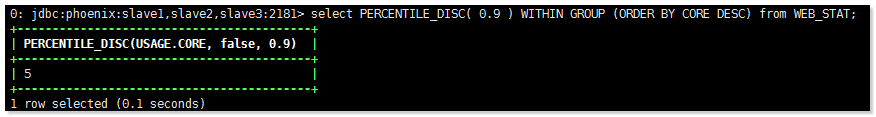### 4.8 PERCENT_RANK

``PERCENT_RANK( 100 ) WITHIN GROUP (ORDER BY X ASC)``
``select PERCENT_RANK(     100 ) WITHIN GROUP (ORDER BY CORE ASC) from WEB_STAT;``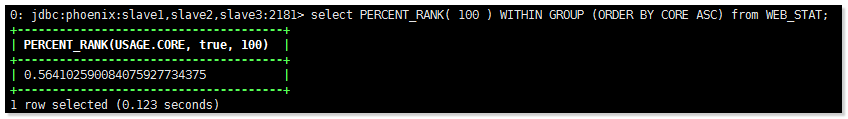### 4.9 FIRST_VALUE

``FIRST_VALUE( name ) WITHIN GROUP (ORDER BY salary DESC)``
``select FIRST_VALUE(     CORE ) WITHIN GROUP (ORDER BY CORE DESC) from WEB_STAT;``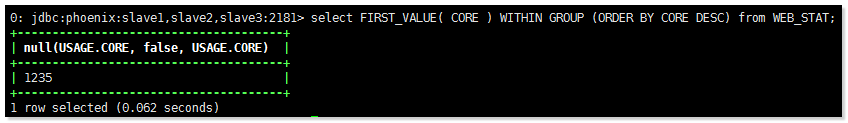### 4.10 LAST_VALUE

``LAST_VALUE( name ) WITHIN GROUP (ORDER BY salary DESC)``
``select LAST_VALUE(     CORE ) WITHIN GROUP (ORDER BY CORE DESC) from WEB_STAT;``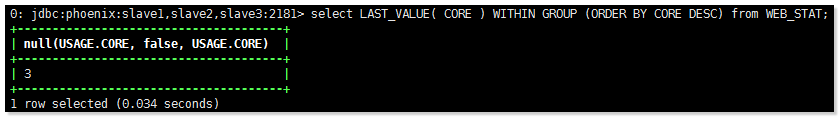### 4.11 NTH_VALUE

``NTH_VALUE( name, 2 ) WITHIN GROUP (ORDER BY salary DESC)``
``select NTH_VALUE(     CORE, 2 ) WITHIN GROUP (ORDER BY CORE DESC) from WEB_STAT;``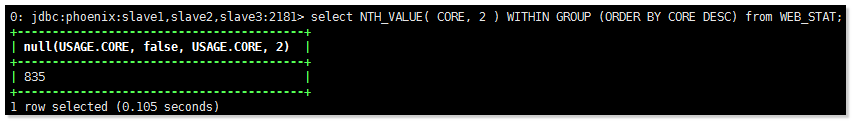### 4.12 STDDEV_POP

``STDDEV_POP( X )``
``select     STDDEV_POP( CORE ) from WEB_STAT;``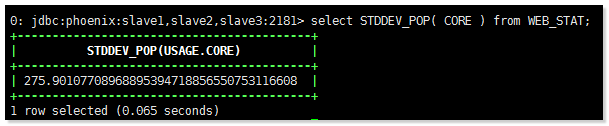### 4.13 STDDEV_SAMP

``STDDEV_SAMP( X )``
``select     STDDEV_SAMP( CORE ) from WEB_STAT;``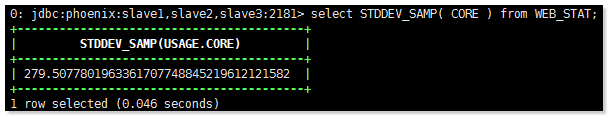## 5. 数组函数

### 5.1 ARRAY_ELEM

``````ARRAY_ELEM(my_array_col, 5)

ARRAY_ELEM(ARRAY[1,2,3], 1)``````
``select     ARRAY_ELEM(ARRAY[1,2,3], 3);``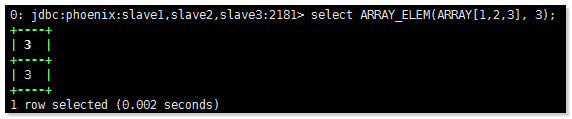### 5.2 ARRAY_LENGTH

``````ARRAY_LENGTH(my_array_col)

ARRAY_LENGTH(ARRAY[1,2,3])``````
``select     ARRAY_LENGTH(ARRAY[1,2,3]);``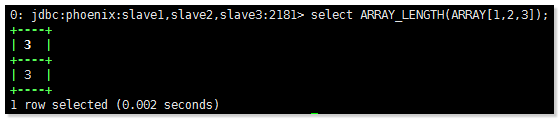### 5.3 ARRAY_APPEND

``````ARRAY_APPEND(my_array_col, my_element_col)

ARRAY_APPEND(ARRAY[1,2,3], 4)  得到数组>> ARRAY[1,2,3,4])``````
``select     ARRAY_APPEND(ARRAY[1,2,3], 4);``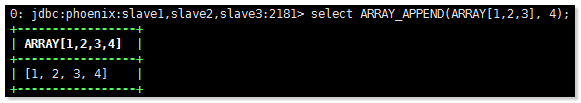### 5.4 ARRAY_PREPEND

``````ARRAY_PREPEND(my_element_col, my_array_col)

ARRAY_PREPEND(0, ARRAY[1,2,3]) 得到数组>> ARRAY[0,1,2,3]``````
``select ARRAY_PREPEND(0, ARRAY[1,2,3]) ;``### 5.5 ARRAY_CAT

``````ARRAY_CAT(my_array_col1, my_array_col2)

ARRAY_CAT(ARRAY[1,2], ARRAY[3,4])  得到数组>> ARRAY[1,2,3,4]``````
``select     ARRAY_CAT(ARRAY[1,2], ARRAY[3,4]);``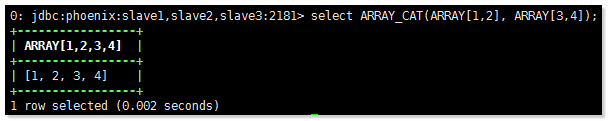### 5.6 ARRAY_FILL

``````ARRAY_FILL(my_element_col, my_length_col)

ARRAY_FILL(1, 3) 得到数组>> ARRAY[1,1,1]``````
``select ARRAY_FILL(1, 3);``### 5.7 ARRAY_TO_STRING

``````ARRAY_TO_STRING(my_array_col, my_delimiter_col, my_null_string_col)

ARRAY_TO_STRING(ARRAY['a','b','c'], ',') evaluates to 'a,b,c'

ARRAY_TO_STRING(ARRAY['a','b',null,'c'], ',') evaluates to 'a,b,c'

ARRAY_TO_STRING(ARRAY['a','b',null,'c'], ',', 'NULL') evaluates to 'a,b,NULL,c'``````
``select     ARRAY_TO_STRING(ARRAY['a','b',null,'c'], ',', 'NULL');``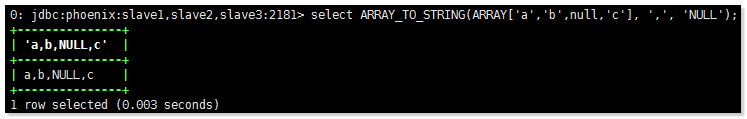### 5.8 ANY

``````1 = ANY(my_array)

10 > ANY(my_array)``````
``select 10 >     ANY(ARRAY[1,2,3]) as result;``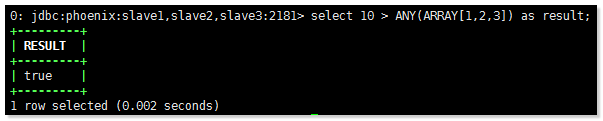``select 10 >     ANY(ARRAY[11,21,13]) as result;``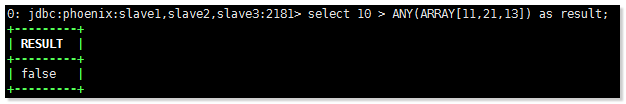### 5.9 ALL

``````1 = ALL(my_array)

10 > ALL(my_array)``````
``select 10 >     ALL(ARRAY[1,2,3]) as result;``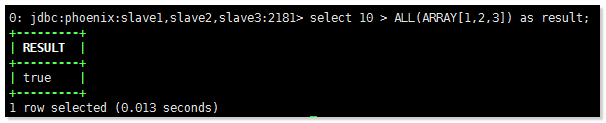``select 10 >     ALL(ARRAY[12,2,3,9]) as result;``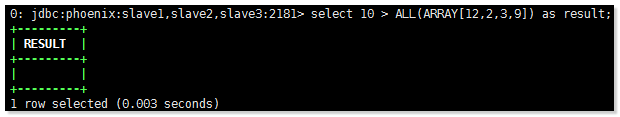## 6. 数学函数

### 6.1 SIGN

``````SIGN(number)

SIGN(1.1)

SIGN(-1)``````
``select SIGN(24);``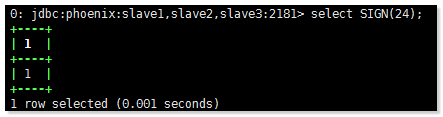### 6.2 ABS

``````ABS(number)

ABS(1.1)

ABS(-1)``````
``select ;``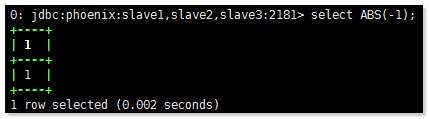### 6.3 SQRT

``````SQRT(number)

SQRT(1.1)``````
``select SQRT(1.1);``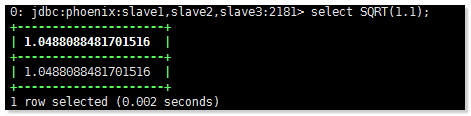### 6.4 CBRT

``````CBRT(number)

CBRT(1.1)

CBRT(-1)``````
``select CBRT(-1);``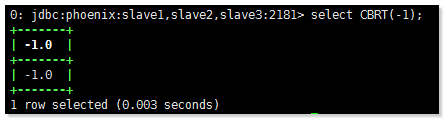### 6.5 EXP

``````EXP(number)

EXP(1.1)

EXP(-1)``````
``select EXP(-1);``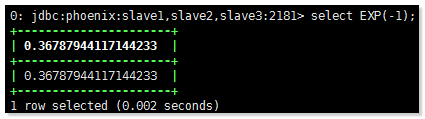### 6.6 POWER

``````POWER(number, number)

POWER(3, 2)

POWER(2, 3)``````
``select POWER(2,     3);``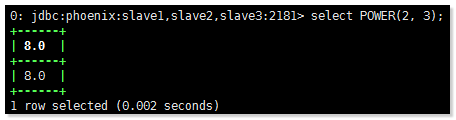### 6.7 LN

``````LN(number)

LN(3)

LN(2)``````
``select LN(2);``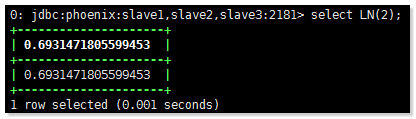### 6.8 LOG

``````LOG(3, 2)

LOG(2, 3)

LOG(2)``````
``select LOG(2);``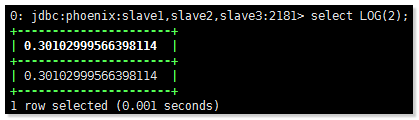## 7. 其他函数

### 7.1 MD5

``MD5(my_column)``
``select MD5(123) as result;``### 7.2 INVERT

``INVERT(my_column)``
``select     INVERT(123) as result;``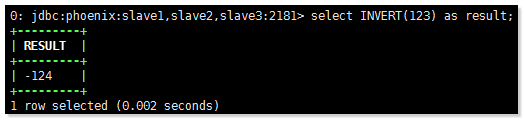### 7.3 ENCODE

``ENCODE(myNumber, 'BASE62')``
``select     ENCODE(123456, 'BASE62') as result;``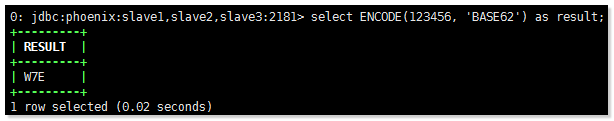### 7.4 DECODE

``DECODE('000000008512af277ffffff8', 'HEX')``
``select     DECODE('000000008512af277ffffff8', 'HEX') as result;``### 7.5 COALESCE

``COALESCE(last_update_date, CURRENT_DATE())``
``select     COALESCE('b', 'a');``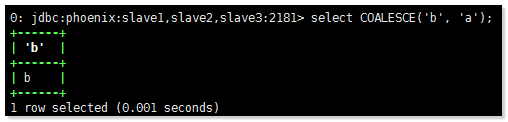### 7.6 GET_BIT

``GET_BIT(CAST('FFFF' as BINARY), 1)``
``Select GET_BIT(CAST('FFFF' as BINARY), 1);``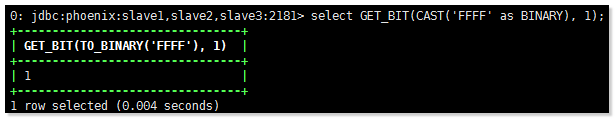### 7.7 GET_BYTE

``GET_BYTE(CAST('FFFF' as BINARY), 1)``
``select     GET_BYTE(CAST('FFFF' as BINARY), 1);``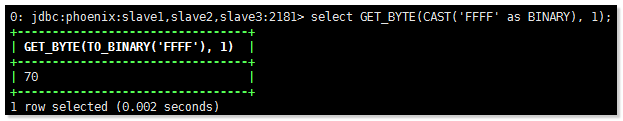### 7.8 OCTET_LENGTH

``OCTET_LENGTH(NAME)``

### 7.9 SET_BIT

``SET_BIT(CAST('FFFF' as BINARY), 1, 61)``
``SELECT     SET_BIT(CAST('FFFF' as BINARY), 1, 61) AS RESULT;``### 7.10 SET_BYTE

``SET_BYTE(CAST('FFFF' as BINARY), 1, 61)``
``SELECT     SET_BYTE(CAST('FFFF' as BINARY), 1, 61) AS RESULT;``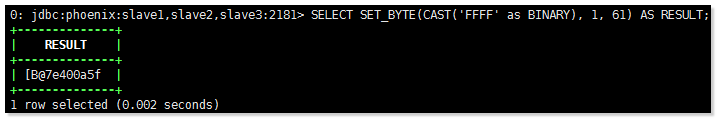上一篇Phoenix 部署与连接HBase
Phoenix 介绍Phoenix 官网：https://phoenix.apache.org/ Phoenix 源码： https://github.com/apache/phoenix HBase是一个 NoSQL 数据库，可存储大量非
2017-03-02Hive 基础教程